# Fractions and percentages

### Term 2 starting in week 7 :: Estimated time: 3 weeks

• Convert fluently between key fractions, decimals and percentages (review)
• Calculate key fractions, decimals and percentages of an amount without a calculator (review)
• Calculate fractions, decimals and percentages of an amount using calculator methods (review)
• Convert between decimals and percentages greater than 100%
• Percentage decrease with a multiplier
• Calculate percentage increase and decrease using a multiplier
• Express one number as a fraction or a percentage of another without a calculator
• Express one number as a fraction or a percentage of another using calculator methods
• Work with percentage change
• Choose appropriate methods to solve percentage problems

For higher-attaining pupils:

• Find the original amount given the percentage less than 100%
• Find the original amount given the percentage greater than 100%
• Choose appropriate methods to solve complex percentage problems

This page should remember your ticks from one visit to the next for a period of time. It does this by using Local Storage so the information is saved only on the computer you are working on right now.

## Lesson Starters

Here are some suggestions for whole-class, projectable resources which can be used at the beginnings of each lesson in this block.

### 1st Lesson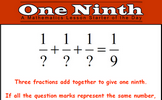#### One Ninth

Investigate three fractions which add together to give one ninth.

### 2nd Lesson#### High Interest

Finding a good personal loan requires an ability to calculate percentage and this page provides some practice.

### 3rd Lesson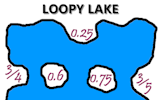#### Loopy Lake

Match the fractions to their decimal equivalents and join them with lines that do not cross.

### 4th Lesson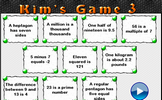#### Kim's Game 3

This is the Maths version of the traditional memory game. Memorise 10 mathematical facts then recall them as they disappear from view.

### 5th Lesson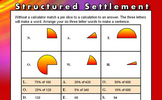#### Structured Settlement

Without a calculator match a a pie slice to a calculation to an answer.

### 6th Lesson#### Table Legs

Learn an unusual times table from the strategic finger moving up and down the 'Table Leg'!

### 7th Lesson#### Odd One Out

From the numbers given, find the one that is the odd one out.

### 8th Lesson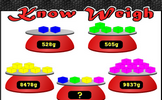### 9th Lesson#### Team Age

Work out who is in which team from the information given.

Some of the Starters above are to reinforce concepts learnt, others are to introduce new ideas while others are on unrelated topics designed for retrieval practice or and opportunity to develop problem-solving skills.

White Rose ResourcesEnd of block assessments provide a quick progress check at the end of each block of learning to make sure students have understood the content covered. This Scheme of Learning was produced by White Rose Maths and is used here with permission granted on 30th June 2021.For All: